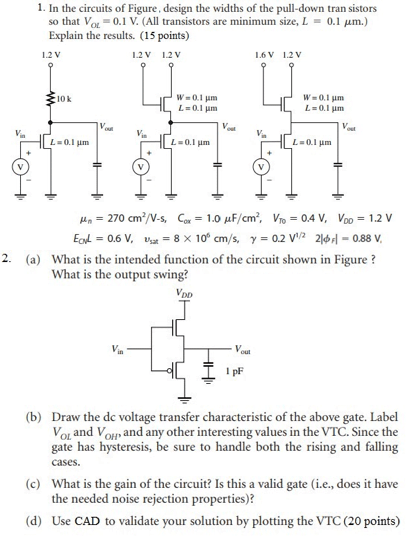# ELEC402 Project 3 Solved

30.00 \$ 15.00 \$

Category:
Click Category Button to View Your Next Assignment | Homework

You'll get a download link with a: . ` zip` solution files instantly, after Payment

## Description

Rate this product– For CAD simulation in Q2 assume VDD = 0.9 V, and use two fingers per transistor.

1. Figure shows a circuit used to measure the effective value of body effect factor (γ) (by measuring VT at different source voltages) and channel length modulation factor (λ) (by measuring Id at different Vds values). Assume in formula for threshold voltage (slide 7 lecture set 4 – MOS basics), 2φF = 0.88 V, and calculate VT0, γ, and λ for a device with 2 fingers. Can you justify the value of γ for the FinFET device you are simulating? Attach your CAD netlist, graphs and measurement data to your answers (25 points)

1. A CMOS inverter in 45 nm technology has a pull-up device that is 8λ:2λ and a pulldown device that is 4λ:2λ. It drives four identical inverters at its output. Compute the inverter delay (use Cg = 2 fF/um) and assume a ramp input. Re-calculate the delay if we have a chain of 4 inverters, i.e. 4 inverters in series (13 pts) Note: 2λ = 45nm